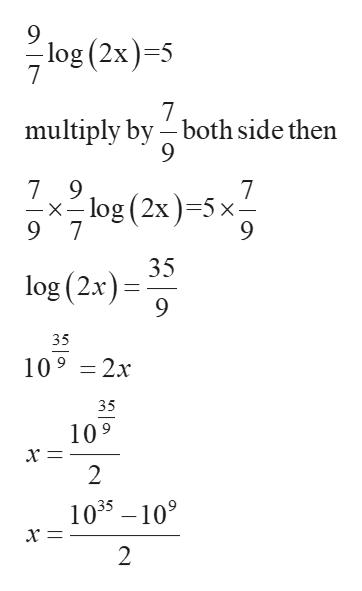Logarithm equations with fractions and how do I simplify a whole # with a fraction exponent

Question

Logarithm equations with fractions and how do I simplify a whole # with a fraction exponent

Step 1

Logarithmic equation with fraction soled by converting fraction logarithm equation into simple logarithm equation after that convert the logarithm equation into exponential equation

Step 2

Example

Take logarithm equation with fraction

Step 3

Now simplifying the...help_outlineImage Transcriptioncloselog (2x)-5 7 7 multiply by both side then 7 9 log (2x) 5 x 9 7 7 -X 35 log (2x) 35 109 2x 35 109 х‑ 2 1035 109 х — 2 fullscreen

Want to see the full answer?

See Solution

Want to see this answer and more?

Our solutions are written by experts, many with advanced degrees, and available 24/7

See Solution
Tagged in

Logarithms